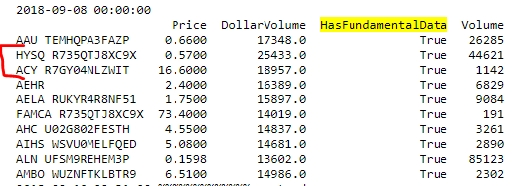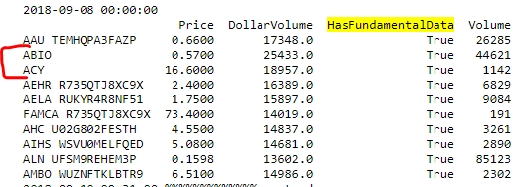Hi, I run this code here to pull the stocks within the 10th and 15th percentile of dolarvolume.

def universe_filter_course(self, coarse_data):
columns = ['Price', 'DollarVolume', 'HasFundamentalData', 'Volume']

data_df = pd.DataFrame.from_records(
[[getattr(s, name) for name in columns] for s in coarse_data],
index=[s.Symbol for s in coarse_data],
columns=columns,
coerce_float=True)

data_df = data_df.query("HasFundamentalData " \
"and (DollarVolume > @data_df.DollarVolume.quantile(.10))" \
"and (DollarVolume < @data_df.DollarVolume.quantile(.15))")
data_df = data_df[:10]
self.Debug(data_df)

Although, when I run the exact same algo twice in a row, I get different results/universes:This is very strange to me as the numbers are the same but the tickers change (???)

Also, as you can see, some tickers have the symbol and the unique ID and others dont.

What am I doing wrong ?

Thanks !